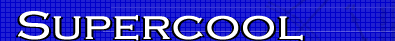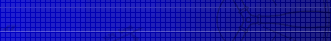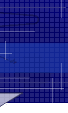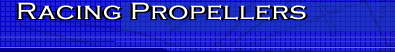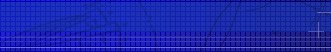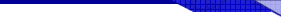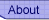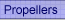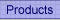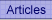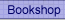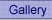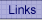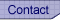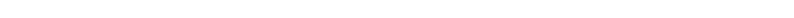﻿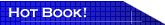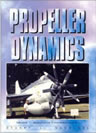Propeller Dynamics Essential reading for model aircraft contest fliers. This is the only book on the market explaining propeller theory in non-mathematical terms. A rattling good read, I know, I wrote it. Read more...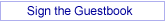## Empirical determination of pitch.

Just how much pitch is required for a given engine/model combination is always a bit of a puzzle. In Goodyear, props range in pitch from 4.5 to 6 inches, which is pretty wild for a standard model class. Since pitch is the advance per revolution, ie, inches moved forward in one turn of the prop, it seems likely pitch may be related to airspeed and engine RPM. Recently New Zealander Bill Sayer was kind enough to send me some data on propeller wind tunnel tests performed in 1934, at the National Physical Laboratory in England. This data was comprehensive, and suggestive of a simple formula for pitch.
Since I cannot pass up a formula without sticking it in my computer, I thought I might share it with readers of VCLN. Given a velocity V in metres per second, and revolutions per second n, then the face pitch P in metres (face pitch is measured to the flat on the bottom of the airfoil at 70% radius) is given by:

P = V / (.9 X n)

Consider a stunter doing 60 MPH with an in-flight RPM of 10000.
Then:

V = 60 * .453 = 27.2 metres per second
n = 10000 / 60 = 166.6 revs per second
P = 27.2/(.9 X 166.6) = .181 metres

and:

P = .181 / .0254 = 7 inches

This seems a little high, but the test propeller at NPL was only 10% thick at the 70% radius. Since thin propellers behave as though they had less pitch, this is probably OK. I recall Steve Rothwell telling me that his props behaved as if there was no slip. That is , the speed could be calculated directly from the face pitch . Professor Larrabee also made a similar comment, in that sections typical of model propellers, being about 13% thick, had a lift coefficient of .5 when the blade was set at zero degrees to the bottom of the airfoil.
Thus Steves formula would be:

P = V / n

and, for the above example,

P = 27.2/ 166.6 = .163 metres

and:

P = .163 / .0254 = 6.4 inches

which also seems reasonable !

Also in the article was an expression for the speed at which zero thrust is generated. This is useful because it gives the maximum possible speed the propeller can provide at a given RPM. If some competitor claims to be going faster than this, you can make with some confidence uncouth suggestions to him.
The formula is bit messy, but if D is the prop diameter in metres then the maximum velocity Vo is given by:

Vo = n X D X (P/D + .151) / (1 - .042 X (P/D))

If the prop in the example above had a diameter of 11" (.279 metres) then:

P/D = .163 / .279 = .584
P/D + .151 = .753
1 -.042 X .584 = .9755

and:

Vo = 166.6 X .279 X .753 / .9755 = 35.88 metres per second or 79 MPH.

The data also revealed a maximum efficiency of this propeller for the above normal flight conditions of 76%. That is,the engine power lost to kinetic energy of the slipstream was 24% of the total. Nothing can be done to improve this, no matter how carefully the propeller is shaped. Thrust is generated by increasing the velocity of the airstream passing through the propeller disc, and as soon as you do this you have lost energy to the slipstream , a real Catch 22 situation !
The data also revealed a very surprising (to me, anyway) situation with respect to the highest efficiency obtainable. The propulsive efficiency of a propeller depends as much on the conditions under which it operates as it does on its actual shape. It appears that the highest efficiency with the pitch-adjustable propeller tested was 86%, and this occurred at the staggering P/D of 2.5 ! So for the 11 X 6.4, you may really want a 4.4 X 6.4 !

Not really, but at higher speeds there is no fundamental reason why the pitch cannot exceed the diameter by a factor of 2 or more: this was certainly the case with the later Schneider trophy racers, and I suggest you look at photos of Rare Bear , or bear Bear (Tu 95), at speed, the pitch is colossal. Do give Steves formula a shot; maybe for your thickness props you may need to do a bit more adjusting. I'm sure readers of VCLN would like to know how you get on.